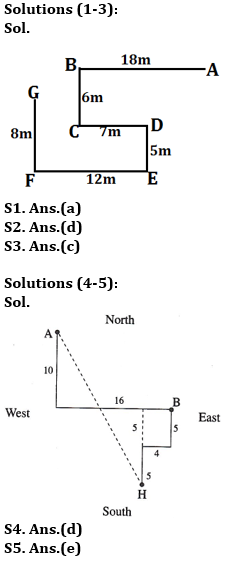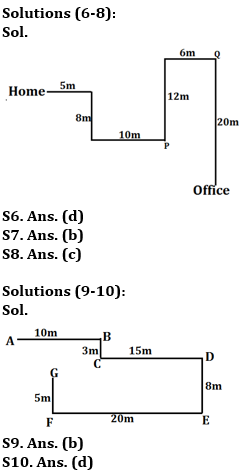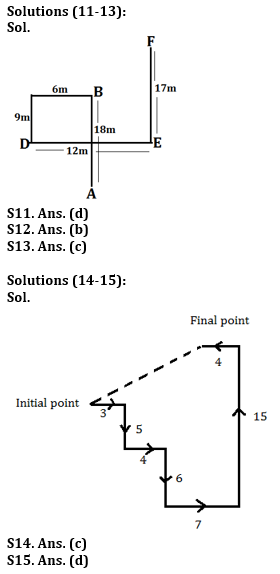Latest Banking jobs   »   Reasoning Ability, Direction Sense Quiz For...

# Reasoning Ability, Direction Sense Quiz For IBPS RRB PO, Clerk Prelims 2021- 28th June

Directions (1-3): Study the following information to answer the given questions.
Point B is 18m west of point A. Point G is 8m north of point F. Point E is 5m south of point D which is 7m east of point C. Point F is 12m west of point E. Point C is 6m south of point B.

Q1. What is the direction of point G with respect to point C?
(a) North-west
(b) South-west
(c) North-east
(d) North
(e) None of these

Q2. What is the shortest distance between point F and point D?
(a) 21m
(b) √15m
(c) 10m
(d) 13m
(e) None of these

Q3. What is the direction of point A with respect to point E?
(a) North
(b) East
(c) North-east
(d) South-west
(e) None of these

Directions (4-5): Study the following information to answer the given questions:
A person starts walking in south direction from point A and covers a distance of 10 meters then he takes a left turn and covers a distance of 16 meters and reaches at point B. From point H, another person starts to walk in north direction and covers a distance of 5 meters then after he takes a right turn and covers a distance of 4 meters and finally takes a left turn and covers a distance of 5 meters and reaches at point B.

Q4. Point H is in which direction from Point A?
(a) East
(b) South
(c) North
(d) South-east
(e) South-west

Q5. Point H is in which direction with respect to Point B?
(a) North-West
(b) North
(c) South-East
(d) South
(e) South- West

A person starts walking in east direction from his home and walks 5m, then takes right turn and walks 8m then he takes left turn and walks 10m to reach at point P. From point P he walks 12m in north direction and then takes a right turn and walks 6m to reach at point Q. Then finally walks in south direction and walks 20m to reach his office.

Q6. What is the direction of his home with respect to point Q?
(a) North-west
(b)West
(c) North-east
(d)South-west
(e) None of these

Q7. What is the shortest distance between point P and his office?
(a) 27m
(b) 10m
(c) 15m
(d) 13m
(e) None of these

Q8. If point R is 4m south of point Q, then what is the shortest distance between his home and point R?
(a) √31m
(b) 14m
(c) 21m
(d) 15m
(e) None of these

Point A is 10m west of point B. Point E is 8m south of point D. Point B is 3m north of point C. Point E is 20m east of point F, which is 5m south of point G. Point C is 15m west of point D.

Q9. What is the direction of point F with respect to point C?
(a)South
(b) South-west
(c)North-east
(d)East
(e) None of these

Q10. What is the shortest distance between point A and Point G?
(a) 19m
(b) √41m
(c) 13m
(d) √61m
(e) None of these

Directions (11-13): Study the following information carefully and answer the question given below:
A person starts walking towards north direction from point A, and walks 18m to reach at point B. From point B he takes two consecutive left turns and walks 6m and 9m respectively and reach at point D. From point D he walks in east direction and walks 12m to reach at point E, then finally takes a left turn and walks 17m to reach point F.

Q11. What is the direction of point E with respect to point A?
(a) East
(b) North-west
(c) South-west
(d) North-east
(e) None of these

Q12. What is the shortest distance between point F to point B?
(a) 15m
(b) 10m
(c) 13m
(d) 16m
(e) None of these

Q13. What is the direction of point D with respect to point F?
(a) South
(b) West
(c) South-west
(d) North-east
(e) None of these

Directions (14-15): Study the following information carefully and answer the given questions.
A person walked for 3km, then took a right turn and walked 5km, then a left turn and walked 4km. and again took a right turn and walked 6km. He then took three consecutive left turns and walked 7 km, 15km and 4 km respectively.

Q14. If the person is now facing west direction, then in which direction did he started walking initially?
(a) North
(b) West
(c) East
(d) South
(e) None of these

Q15. What is the shortest distance between the initial and final point of the person? (Apply the condition given in question number 9).
(a) √41 km
(b) √29 km
(c) 3 √43 km
(d) 2√29 km
(e) None of these

ractice More Questions of Reasoning for Competitive Exams:

###### Study Plan for IBPS RRB PO/Clerk Prelims 2021

Solutions